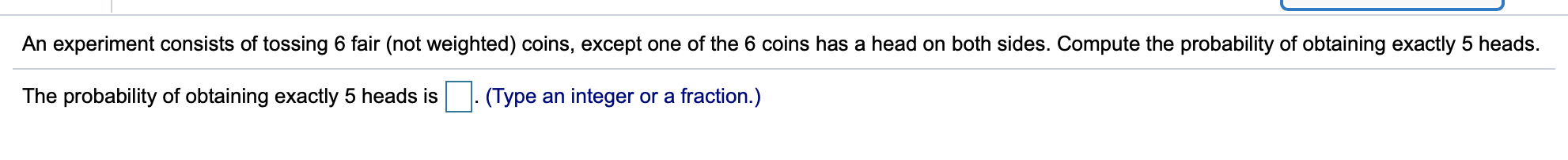# An experiment consists of tossing 6 fair (not weighted) coins, except one of the 6 coins has a head on both sides. Compute the probability of obtaining exactly 5 headsThe probability of obtaining exactly 5 heads is(Type an integer or a fraction.)

Questionhelp_outlineImage TranscriptioncloseAn experiment consists of tossing 6 fair (not weighted) coins, except one of the 6 coins has a head on both sides. Compute the probability of obtaining exactly 5 heads The probability of obtaining exactly 5 heads is (Type an integer or a fraction.) fullscreen
check_circleExpert Solution

The total number of trials is 6. It is given that one of the 6 coins has a head on both sides.

On...

### Want to see the full answer?

See Solution

#### Want to see this answer and more?

Solutions are written by subject experts who are available 24/7. Questions are typically answered within 1 hour*

See Solution
*Response times may vary by subject and question
Tagged in

### Basic Probability